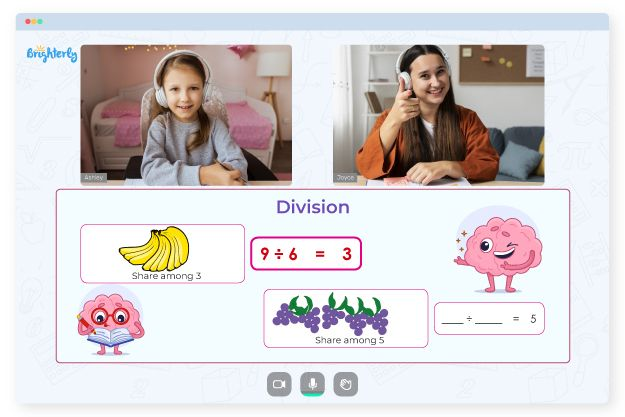# Multiplication and Division Fact Families Worksheets

There are fact families in multiplication and division which your kids must learn to work with. Children should know how to solve addition, subtraction, and each equation around the fact families. Using fact families multiplication and division worksheets, you can easily teach them how to work with this math concept. Multiplication and division facts worksheets help kids remember the formulas and equations they need to solve these problems.

## Benefits of fact family multiplication and division worksheets

Kids often need a visual representation of what kind of questions they will have to deal with, and multiplication division fact family worksheets explain the topic easily and prepare them for practice tests ahead.

Math for Kids

Is Your Child Struggling With Math?
1:1 Online Math TutoringYou can download as many fact family worksheets multiplication and division pdf as you want from math websites on the internet. Get printable ones so that your kids can stay engaged the entire time.### Multiplication and Division Fact Families Worksheets PDF

Multiplication Division Fact Family Worksheets### Multiplication and Division Fact Families Worksheets PDF

Fact Family Worksheets Multiplication And Division PDF### Multiplication and Division Fact Families Worksheets PDF

Fact Family Multiplication and Division Worksheets### Multiplication and Division Fact Families Worksheets PDF

Fact Families Multiplication and Division Worksheets

Problems with Division?• Is your child finding division concepts challenging?
• An online tutor could provide the necessary assistance.

Does your child struggle with understanding the concept of division? Try lessons with an online tutor.